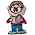## Wednesday, March 25, 2009

### Fill in the Question

What number should replace the ?

9  4  6 11
3  6  7  4
5  ?  4  1
11 6 3  8

1.The answer is 8.

Going along each row, the fourth number is the sum of the first and third numbers, minus the second number. So for each row we have:
9 + 6 - 4 = 11
3 + 7 - 6 = 4
5 + 4 - 8 = 1
11 + 3 - 6 = 8

The same pattern holds going down the columns:
9 + 5 - 3 = 11
4 + 8 - 6 = 6
6 + 4 - 7 = 3
11 + 1 - 4 = 8

2.You have the pattern down and the answer is 8.

I would have said take the first and third number and add it to get get the same number as when you add the second and fourth number. This is true across each column and each row. For instance in the first row, 9+6 = 15; 4+11 =15.

Leave your answer or, if you want to post a question of your own, send me an e-mail. Look in the about section to find my e-mail address. If it's new, I'll post it soon.

Please don't leave spam or 'Awesome blog, come visit mine' messages. I'll delete them soon after.

Enter your Email and join hundreds of others who get their Question of the Day sent right to their mailbox

The Lamplight Manor Puzz 3-D